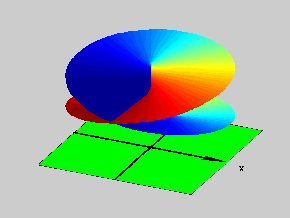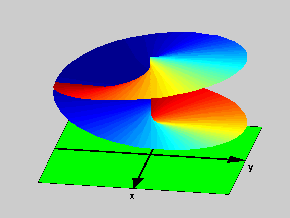18.04 | Fall 1999 | Undergraduate

# Complex Variables with Applications

Study Materials

## Riemann Surfaces: The Square Root

(All images created with MATLAB® software)

Click on picture for more information and an image of higher resolution.

The square root provides another simple example of a Riemann Surface. The pictures here show two views of this surface, whose construction is explained next.

Consider again a Complex Plane cut along the negative real axis, just as in the case of the logarithm. However: instead of having infinitely many possible branches on this cut plane, we have now only two. These are given by the principal value of the square root and its negative (the principal value of the square root is defined in exactly the same fashion as the principal value of the cubic root was  defined earlier). It would seem that this should lead to a surface simpler than that of the logarithm, but it actually does not.Figure 1: One view of a square root Riemann Surface

The reason is as follows: when joining the two cut planes to make the surface, we have to join the lower lip of the cut in one plane with the upper in the other (and conversely). But this cannot be done within three dimensional space, since once we join one pair of lips, the members of the other pair end up on different sides of the surface. To join this second pair, we must go into the “fourth dimension” (this is exactly the same sort of difficulty you would find if you try to make a knot in a curve while staying on a plane). What this all means is that: the Riemann Surface for the square root is an object in four dimensional space. In the pictures we have color coded the surface: at the (inevitable in three dimensions) crossings each of the two sheets that cross have a clearly distinct coloring (this is the best we could do with our 4-D coloring pens broken).Figure 2: Another view of a square root Riemann Surface